# ISEE Upper Level Quantitative : How to find mean

## Example Questions

### Example Question #101 : Data Analysis

John's grade in his economics class is the mean of his best five test scores out of the six tests he takes.

John's first five test scores are: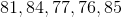Which is the greater quantity?

(a) The lowest score John must take to achieve a score of at least a score offor the term

(b)(a) and (b) are equal.

(a) is greater.

(b) is greater.

It is impossible to tell from the information given.

(b) is greater.

Explanation:

John's current point sum is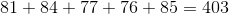.

Even if John achieves a score lower than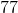on his sixth test, he will have at least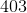points and a minimum mean of at least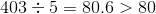. He can even score zero points on his last test and keep his average above what he wants, making (b) greater.

### Example Question #12 : How To Find Mean

A student's grade in Professor Jackson's Shakespeare class is the mean of his or her four best test scores out of five.

Craig outscored Jerry on the first test by 9 points and on the fifth test by 5 points. Jerry outscored Craig by 6 points on the second test and by 8 points on the fourth. Their scores were identical on the third.

Which is the greater quantity?

(a) and (b) are equal.

(b) is greater.

It is impossible to tell from the information given.

(a) is greater.

It is impossible to tell from the information given.

Explanation:

Let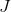stand for Jerry's total score after his lowest test is thrown out.

We need to compare the sums after the lowest test for each student is disregarded, since each will be divided by the four tests. But it is not known which test will be thrown out for each student.

If, for example, the first test is thrown out for both Craig and Jerry, Craig's total will be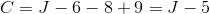,

and Jerry's score will be higher.

If the second test is thrown out for both Craig and Jerry, Craig's total will be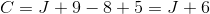,

and Craig's score will be higher.

### Example Question #13 : How To Find Mean

A student's grade in Professor Kalton's abstract algebra class is the mean of his or her five test scores.

Philip outscored Kellie on the first test by 8 points and on the second test by 5 points. They scored the same on the third test. Kellie outscored Philip by 7 points on the fourth test and by 6 points on the fifth.

Which is the greater quantity?

(a) is greater.

(a) and (b) are equal.

It is impossible to tell from the information given.

(b) is greater.

(a) and (b) are equal.

Explanation:

You do not need to take the two means; just compare the sums, since each will be divided by 5.

Let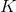be Kellie's total points. Then since Philip outscored Kellie by 8 points and 5 points on two tests and scored fewer than Kellie by 7 points and 6 points on two tests, Philip's score is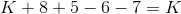.

Philip and Kellie scored the same number of points, making their mean test scores the same.

### Example Question #14 : How To Find Mean

Katie's grade in her Shakespeare class is the mean of her best five test scores out of six tests taken.

Her test scores are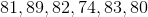.

Which is the greater quantity?

(b)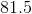(a) and (b) are equal.

(a) is greater.

(b) is greater.

It is impossible to tell from the information given.

(a) is greater.

Explanation:

Take the sum of all of her test scores except for her lowest and divide that by the number of test scores included.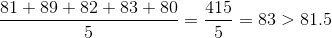### Example Question #111 : Data Analysis

Consider the following set of numbers: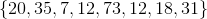Quantity A: Median of the set

Quantity B: Mean of the set

The relationship cannot be determined from the information given.

Quantity A is greater.

The two quantities are equal.

Quantity B is greater.

Quantity B is greater.

Explanation:

The median of the set of numbers is determined by arranging the numbers in numerical order and finding the middle number. In this case there are two middle numbers,and, so we find the average of those numbers, which gives us.

The mean is found by dividing the sum of elements by the number of elements in the set: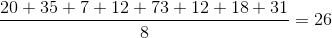Quantity B is larger.

### Example Question #16 : How To Find Mean

Compareand: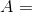The average of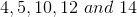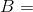The average of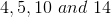It is not possible to determine which quantity is greater.

The quantity ofis greater thanThe two quantities are equal.

The quantity ofis greater thanThe quantity ofis greater thanExplanation:

The average of a list of terms can be found as follows: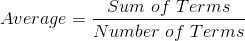So we can write: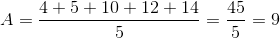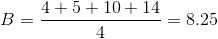Sois greater than### Example Question #17 : How To Find Mean

Which one is greater: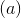The mean of the data set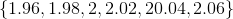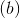The mean of the data set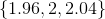is greater

It is not possible to tell from the information givenis greaterandare equalis greater

Explanation:

Mean of a data set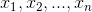is the sum of the data set values divided by the number of data: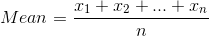So we have: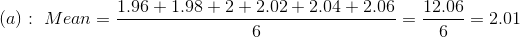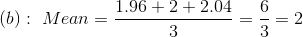So the mean of the first data set is greater.

### Example Question #18 : How To Find Mean

Which one is greater:The mean of the data set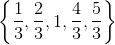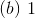It is not possible to tell from the information givenandare equalis greateris greaterandare equal

Explanation:

Mean of a data setis the sum of the data set values divided by the number of data:So we have: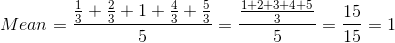### Example Question #19 : How To Find Mean

Which is greater:The mean of the data set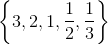is greateris greaterandare equal

It is not possible to tell from the information givenis greater

Explanation:

Mean of a data setis the sum of the data set values divided by the number of data:So we have: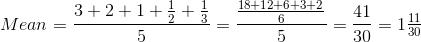So the mean of the data set is greater than### Example Question #20 : How To Find Mean

Which one is greater:The mean of the data set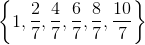is greaterandare equal

It is not possible to tell from the information givenis greateris greater

Explanation:

Mean of a data setis the sum of the data set values divided by the number of data:So we have: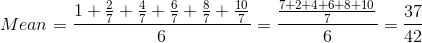So the mean of the data set is smaller than.